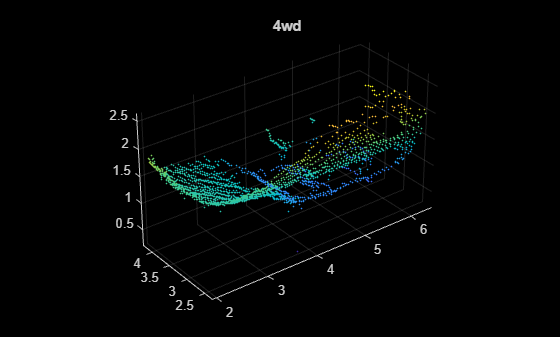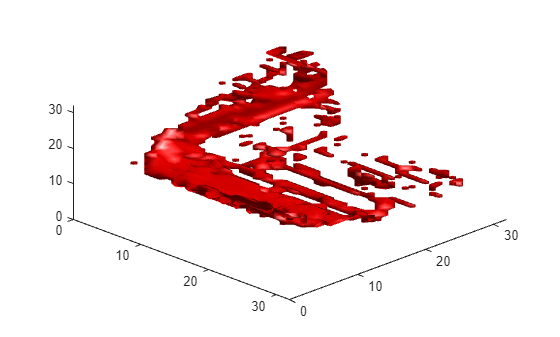# Encode Point Cloud Data For Deep Learning

When using convolutional neural networks with point cloud data, certain core operations like convolution require input data that is regularly sampled spatially. The irregular spatial sampling of point cloud and lidar data must be transformed into some regularly sampled structure at some point in the preprocessing pipeline. There are many different approaches to how point cloud data is transformed into a dense, gridded structure . This example demonstrates a simple approach known as voxelization.

### Voxelization of Point Cloud Data

Start by defining a datastore for working with the Sydney Urban Objects Dataset.

```dataPath = downloadSydneyUrbanObjects(tempdir); ds = loadSydneyUrbanObjectsData(dataPath);```

Obtain sample output data from datastore.

```data = preview(ds); disp(data)```
``` {1×1 pointCloud} {[4wd]} ```

View sample output data from datastore

```figure ptCloud = data{1}; pcshow(ptCloud); label = string(data{2}); title(label);```Use the `pcbin` function to define a desired regular 3-D gridding of the coordinate system of an input `pointCloud` object. Use `pcbin` to also return an output cell array that contains spatial bin locations for each point in the input `pointCloud`. In this case, the input `pointCloud` is binned in a [32,32,32] size output grid that spans the XLimits,YLimits, and ZLimits of the input `pointCloud`.

```outputGridSize = [32,32,32]; bins = pcbin(data{1},outputGridSize);```

Each cell in `bins` contains the indices of the points in `ptCloud`.`Location` that fall in a particular point location. The MATLAB function `cellfun` can be used to define common encodings of point cloud data using `bins` as input.

`occupancyGrid = cellfun(@(c) ~isempty(c),bins);`

Define a 3-D occupancy grid which is true for grid locations that are occupied by at least one point and false otherwise.

```figure; p = patch(isosurface(occupancyGrid,0.5)); view(45,45); p.FaceColor = 'red'; p.EdgeColor = 'none'; camlight; lighting phong```### Transform Datastore to Apply Point Cloud Encoding to Entire Dataset

Use the `transform` function of datastore to apply a simple occupancy grid encoding to every observation in an input datastore. The `formOccupancyGrid` function, which is included in the supporting functions section, uses the exact same approach shown above with `pcbin`.

```dsTransformed = transform(ds,@formOccupancyGrid); exampleOutputData = preview(dsTransformed); disp(exampleOutputData);```
``` {32×32×32 logical} {[4wd]} ```

The resulting datastore, `dsTransformed`, can be passed to deep learning interfaces including `trainNetwork` and `DataLoader` for use in training deep neural networks.

### References

 Maturana, D. and Scherer, S., VoxNet: A 3D Convolutional Neural Network for Real-Time Object Recognition, IROS 2015.

 AH Lang, S Vora, H Caesar, L Zhou, J Yang, O Beijbom, PointPillars: Fast Encoders for Object Detection from Point Clouds, CVPR 2019

 Charles R. Qi, Hao Su, Kaichun Mo, Leonidas J. Guibas, PointNet: Deep Learning on Point Sets for 3D Classification and Segmentation, CVPR 2017

### Supporting Functions

```function datasetPath = downloadSydneyUrbanObjects(dataLoc) if nargin == 0 dataLoc = pwd(); end dataLoc = string(dataLoc); url = "http://www.acfr.usyd.edu.au/papers/data/"; name = "sydney-urban-objects-dataset.tar.gz"; if ~exist(fullfile(dataLoc,'sydney-urban-objects-dataset'),'dir') disp('Downloading Sydney Urban Objects Dataset...'); untar(url + name,dataLoc); end datasetPath = dataLoc.append('sydney-urban-objects-dataset'); end function ds = loadSydneyUrbanObjectsData(datapath,folds) % loadSydneyUrbanObjectsData Datastore with point clouds and % associated categorical labels for Sydney Urban Objects dataset. % % ds = loadSydneyUrbanObjectsData(datapath) constructs a datastore that % represents point clouds and associated categories for the Sydney Urban % Objects dataset. The input, datapath, is a string or char array which % represents the path to the root directory of the Sydney Urban Objects % Dataset. % % ds = loadSydneyUrbanObjectsData(___,folds) optionally allows % specification of desired folds that you wish to be included in the % output ds. For example, [1 2 4] specifies that you want the first, % second, and fourth folds of the Dataset. Default: [1 2 3 4]. if nargin < 2 folds = 1:4; end datapath = string(datapath); path = fullfile(datapath,'objects',filesep); % For now, include all folds in Datastore foldNames{1} = importdata(fullfile(datapath,'folds','fold0.txt')); foldNames{2} = importdata(fullfile(datapath,'folds','fold1.txt')); foldNames{3} = importdata(fullfile(datapath,'folds','fold2.txt')); foldNames{4} = importdata(fullfile(datapath,'folds','fold3.txt')); names = foldNames(folds); names = vertcat(names{:}); fullFilenames = append(path,names); ds = fileDatastore(fullFilenames,'ReadFcn',@extractTrainingData,'FileExtensions','.bin'); end function dataOut = extractTrainingData(fname) [pointData,intensity] = readbin(fname); [~,name] = fileparts(fname); name = string(name); name = extractBefore(name,'.'); labelNames = ["4wd","bench","bicycle","biker",... "building","bus","car","cyclist","excavator","pedestrian","pillar",... "pole","post","scooter","ticket_machine","traffic_lights","traffic_sign",... "trailer","trash","tree","truck","trunk","umbrella","ute","van","vegetation"]; label = categorical(name,labelNames); dataOut = {pointCloud(pointData,'Intensity',intensity),label}; end function [pointData,intensity] = readbin(fname) % readbin Read point and intensity data from Sydney Urban Object binary % files. % names = ['t','intensity','id',... % 'x','y','z',... % 'azimuth','range','pid'] % % formats = ['int64', 'uint8', 'uint8',... % 'float32', 'float32', 'float32',... % 'float32', 'float32', 'int32'] fid = fopen(fname, 'r'); c = onCleanup(@() fclose(fid)); fseek(fid,10,-1); % Move to the first X point location 10 bytes from beginning X = fread(fid,inf,'single',30); fseek(fid,14,-1); Y = fread(fid,inf,'single',30); fseek(fid,18,-1); Z = fread(fid,inf,'single',30); fseek(fid,8,-1); intensity = fread(fid,inf,'uint8',33); pointData = [X,Y,Z]; end function dataOut = formOccupancyGrid(data) grid = pcbin(data{1},[32 32 32]); occupancyGrid = cellfun(@(c) ~isempty(c),grid); label = data{2}; dataOut = {occupancyGrid,label}; end```